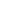Add and subtract tens from a multiple of 10.

EngageNY 60 min(s)
In this lesson, students represent the addition of ten more with concrete objects and number bonds. (For example, they represent 4 as 3 and 1 together; 40 as "3 tens" and "1 ten" together; and 40 as 30 and 10 together.) After creating number bonds for several examples, they notice that only the unit has changed (for example, 3 bananas + 1 banana = 4 bananas, just as 3 tens + 1 ten = 4 tens). They also see that this relationship is present even when adding more than 1 ten (for example, 2 tens + 2 tens = 4 tens, just as 2 + 2 = 4) and decomposing to subtract (for example, 4 tens - 3 tens = 1 ten, just as 4 - 3 = 1). In the process, they learn that arrow notation is used to show the addition or subtraction of an amount, regardless of whether the number is increasing (adding) or decreasing (subtracting). This provides an important foundation for applying the "make ten" strategy in upcoming lessons.
*Teacher Advisor is 100% free.

Teacher Advisor uses cookies and other tracking technologies for performance, analytics, and marketing purposes.

By using this website, you accept this use. Learn more about these technologies in the Privacy policy.